nextnano.com  nextnano³  Download | Search | Copyright | Publications  * password protected nextnano³ software1D nin Si resistor

# nextnano3 - Tutorial

## n-i-n Si resistor

Author: Stefan Birner

If you want to obtain the input file that is used within this tutorial, please submit a support ticket.
`-> Si_nin_resistor_classical_1D_nn3.in / *_nnp.in - `input file for the nextnano3 and nextnano++ software```    Si_nin_resistor_quantum_1D_nn3.in   / *_nnp.in - ```input file for the nextnano3 and nextnano++ software

This tutorial is based on the example presented on p. 43 in Stefan Hackenbuchner's PhD thesis, TU Munich (2002) and on the following paper:

Towards fully quantum mechanical 3D device simulation
M. Sabathil, S. Hackenbuchner, J.A. Majewski, G. Zandler, P. Vogl
Journal of Computational Electronics 1, 81 (2002)

## n-i-n Si resistor

`-> Si_nin_resistor_classical_1D_nn3.in`

Here, we illustrate our method for calculating the current by studying simple one-dimensional examples that we can compare to full Pauli master equation results. Our method amounts to calculating the electronic structure of a device fully quantum mechanically, yet employing a semi-classical scheme for the evaluation of the current. As we shall see, the results are close to those obtained by the full Pauli master equation provided we limit ourselves to situations not too far from equilibrium.

We consider a one-dimensional 300 nm Si-based n-i-n resistor at room temperature, where "n-i-n" stands for "n-doped / intrinsic / n-doped". The intrinsic region and the n-doped regions are each 100 nm wide.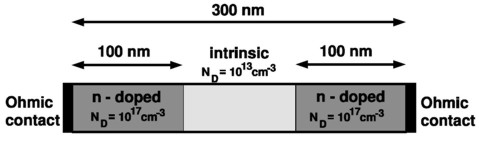The figure shows the geometry of the n-i-n Si resistor.

The n-doped regions at the left and right sides are doped with a doping concentration of ND = 1 x 1017 cm-3.
The intrinsic region in the center of the device has a background concentration of ni = 1 x 1013 cm-3 (according to PhD thesis of S. Hackenbuchner, p. 43) (why? compare with value of ni some lines below! Because of Fermi-Dirac statistics or because of other valleys' contribution?). This value is calculated by nextnano³ automatically and does not have to be entered in the input file. Assuming Maxwell-Boltzmann statistics, the intrinsic carrier concentration ni is given by:

ni = (Nc Nv)1/2 exp (- Egap / (2 kB T))

T = 300 K; T: temperature

kB T =  eV (at room temperature)

Egap = 1.095 eV; Egap: band gap energy of Si at 300 K

Nc = 2.738*1019 cm-3 (see Tutorial "I-V characteristics of an n-doped Si structure")

Nv = 1.138*1019 cm-3 (see Tutorial "I-V characteristics of an n-doped Si structure")

Note: Using this equation, one obtains ni = 1.12*1010 cm-3.

For a more detailed discussion of this equation (including Fermi-Dirac statistics), please read the description in Tutorial "I-V characteristics of an n-doped Si structure".

At both ends of the device there are ohmic contacts.

The conductivity electron mass is given by

me*cond = 3 / (1/0.916 + 2/0.19) m0 = 0.258 m0

whereas the DOS electron effective mass is given by

me*DOS = (0.916·0.192)1/3 m0 = 0.321m0.

The static dielectric constant is given by epsilon = 11.7.

For the donors we assumed an ionization energy of 0.015 eV as well as a degeneracy factor of 2.

For the mobility which should depend on the electric field and on the concentration of ionized impurities we assumed``` mobility-model-simba-2 ```and used the following parameters:

```  \$mobility-model-simba           !                                   !    material-name       = Si       ! ``` taken from the SIMBA manual```                                   !    n-alpha-doping      = 0.73     ! []    n-N-ref-doping      = 1.072e17 ! [1/cm3]    n-mu-min            = 55.2     ! [cm2/Vs]    n-mu-doping         = 1374.0   ! [cm2/Vs]    n-gamma-temp        = 1.5      ! []    n-E0-saturation     = 8.0e3    ! [V/cm]    n-T0-E-saturation   = 300.0    ! [K]    n-temp-dependence-E = 0.0      ! [V/Kcm]    n-alpha-E           = 2.0      ! []    n-beta-E            = 0.5      ! []    n-v0-saturation     = 1.03e7   ! [cm/s]    n-temp-dependence-v = 0.0      ! [cm/Ks]    n-kappa-v           = 2.0      ! []    n-ET-perpendicular  = 64.970e3 ! [V/cm]```

The electron density in nextnano³ can be calculated in two ways:

• classical density (Thomas-Fermi approximation)
• quantum mechanical density (local quasi-Fermi levels): The charge density is calculated for a given applied voltage by assuming the carriers to be in local equilibrium that is characterized by energy-band dependent local quasi-Fermi levels EF(x) (i.e. in the simplest case, one for holes and one for electrons)
These local quasi-Fermi levels are determined by global current conservation div j = 0, where the current is assumed to be given by the semi-classical relation j = µ(x) n(x) grad EF(x) where µ(x) is the electron mobility which is determined in our example from``` mobility-model-simba-2```.
The carrier wave functions and energies are calculated by solving the single-band Schrödinger-Poisson equation self-consistently. The open system is mimicked by using mixed Dirichlet and Neumann boundary conditions (Fischetti (1998), Lent and Kirkner (1990), Frensley (1992)) at ohmic contacts. (Note: The feature of using "mixed" boundary conditions for the Schrödinger equation is not supported any more.) The Schrödinger, Poisson and current continuity equations are solved iteratively. As a preparatory step, the built-in potential is calculated for zero applied bias by solving the Schrödinger-Poisson equation self-consistently employing a predictor-corrector approach and setting to zero the electric field at the ohmic contacts. For applied bias, the Fermi level and the potential at the contacts are then shifted according to the applied potential which fixes the boundary conditions.
The main iteration scheme itself consists of two parts:
- In the first part, the wave functions and potential are kept fixed and the quasi-Fermi are calculated self-consistently from the current continuity equation.
- In the second part, the quasi-Fermi levels are kept constant, and the density and the potential are calculated self-consistently from the Schrödinger and Poisson equations.

### Classical and quantum mechanical electron densities at equilibrium, i.e. applied bias = 0 V

Now let us first have a look at the electron densities for the cases of
a) classical and
b) quantum mechanical calculations.

The electron density is the sum over all three valleys (Gamma point, L point and X point (or Delta for Si) in the Brillouin zone) whereas for Si the dominant valley is the X valley which is sixfold degenerate (or twelvefold degenerate including spin degeneracy). Thus we solve Schrödinger's equation only in the X valley and take for the other valleys the classical density only.

For b) we have to choose appropriate boundary conditions where we choose "mixed". (Note: The feature of using "mixed" boundary conditions for the Schrödinger equation is not supported any more.) For comparison we also show the results of Dirichlet and Neumann boundary conditions although we do not recommend the latter for current calculations.
Dirichlet boundary conditions lead to a problem, namely the density at the contacts is then zero (`-> `no current).

``` \$quantum-model-electrons   ...   boundary-condition-100 = mixed     ! ```mixed = Dirichlet + Neumann (i.e. the Schrödinger equation has to be solved twice) (not supported any more)```   boundary-condition-100 = Dirichlet ! ``` Dirichlet```   boundary-condition-100 = Neumann   ! ```Neumann

To switch off the current calculation, we have to choose:
``` \$current-cluster   ...   deactivate-cluster = yes  ! ```flag to switch off current clusters

In the following figure we compare for 0 V applied bias, i.e. for equilibrium where no current flows, the classical and the quantum mechanical electron densities.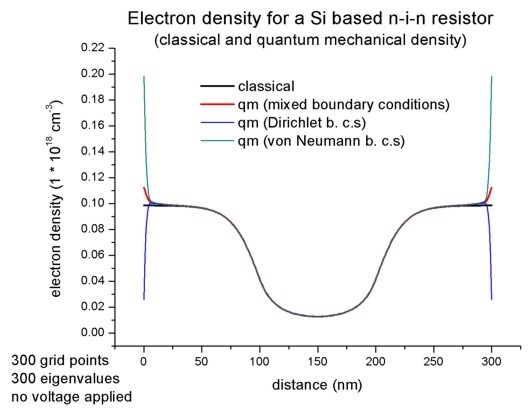Classical and quantum mechanical electron densities for the n-i-n resistor. Dirichlet boundary conditions force the wave function to be zero at the boundaries and thus the electron density is zero as well. Thus to get a meaningful and physical electron density at the boundaries we have to choose "mixed" boundary conditions. (Note: The feature of using "mixed" boundary conditions for the Schrödinger equation is not supported any more.)

### Current-voltage characteristics of the Si n-i-n resistor

Now we vary the applied bias in steps of 0.05 V (```-> \$voltage-sweep```) and solve the drift-diffusion equations without taking into account quantum mechanical densities, i.e. for a classical simulation.
In addition to the above mentioned``` mobility-model-simba-2 ```which depends on doping and on the electric field, we compare our results to the ones obtained with a constant mobility model (µ = 1417 cm²/Vs).

```  \$mobility-model-constant    material-name     = Si    n-mu-lattice-temp = 1417.0 ! [cm²/Vs]  ```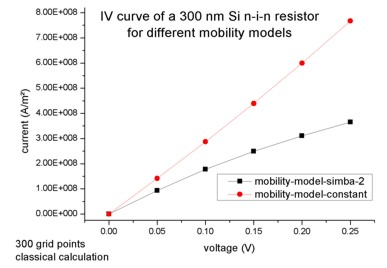IV characteristics of the 300 nm Si n-i-n resistor for two different mobility models (classical simulations).

The conduction band edges and the Fermi levels (i.e. chemical potentials) for the electrons at different applied voltages are plotted in this figure: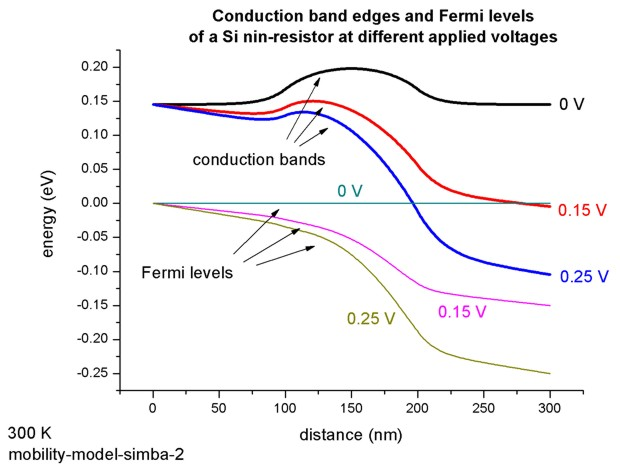### Quantum mechanical calculations

`-> Si_nin_resistor_quantum_1D_nn3.in`

As one may expect, true quantum mechanical effects play little role in this case and both the nextnano³ (i.e. the semi-classical drift-diffusion) and the Pauli master equation approach yield practially identical results for the density and conduction band edge energies (i.e. for the electrostatic potential). We would like to point out that this good agreement is a nontrivial finding, as we calculate the density quantum mechanically with self-consistently computed local quasi-Fermi levels rather than semi-classically.

The following figure shows for an applied bias of 0.25 V the conduction band edge energies and the electron densities. One can see that our results agree very well with the solution of the Pauli master equation (M.V. Fischetti, J. Appl. Phys. 83, 270 (1998)). Fischetti obtains for the current density 6.8 * 104 A/cm² whereas we obtain 3.65 * 104 A/cm² by using a (semi-)classical drift-diffusion model. However, we note that the current is directly proportional to the mobility in our model, i.e. changing the mobility therefore changes the value of the current but does not affect the electron density or potential profile (is this really true?). If we had chosen a constant mobility of µ = 1417 cm²/Vs then the current at 0.25 V applied bias had been 7.67 * 104 A/cm² (compare with I-V characteristics above).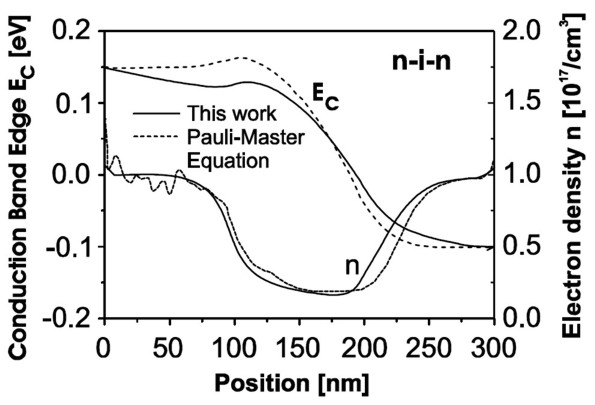The figure shows the calculated conduction band edges Ec and the electron densities n of the n-i-n structure as a function of position inside the structure. The results obtained from the Pauli master equation (Fischetti (1998), dashed lines) are compared to our quantum mechanical results (full lines).

First one could think that the good agreement between the two approaches is trivial, as quantum mechanical effects do not play any role in this example. However, we want to stress that the electron density was calculated fully quantum mechanically with self-consistent local quasi-Fermi levels.

Conclusion

Here, we demonstrated our approach to calculate the electronic structure in nonequilibrium where we combine the stationary solutions of the Schrödinger equation with a semi-classical drift-diffusion model. For the electrostatic potential and the charge carrier density, the method leads to a very good agreement with the more rigorous Pauli master equation approach. In addition, the current can also be described accurately.